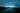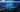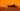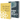# React Render Props

by Robin WieruchThe concept of children as a function or child as a function, also called render prop in general, is one of the advanced patterns in React (next to ). The components which implement this pattern could be called render prop components. In this tutorial, you will learn everything about React's render prop pattern. Along the way, you will implement a currency converter in React, see how the render prop pattern can be compensated with alternative patterns in React too, but then how it can be solved with a render prop component. Afterward, the usage of the render prop pattern should be clear and there shouldn't be any confusion about children as a function, render prop or render prop components left.

## The case for React Render Prop

Let's get started with the implementation of the currency converter in order to learn about React's render prop pattern. If you want to follow in your own editor/IDE, you can use create-react-app to get your application up and running. Then the only file you need to touch will be the src/App.js file:

`import React, { Component } from 'react'; const App = () => (  <div>    <h1>Currency Converter</h1>  </div>); export default App;`

Firstly, let's implement a new React component next to the App component where you can increment (and decrement) a number. In this case, the abstract number will be the amount of US Dollar which should be converted to other currencies later on:

`const App = () => <Amount />; class Amount extends Component {  constructor(props) {    super(props);     this.state = {      amount: 0,    };  }   onIncrement = () => {    this.setState(state => ({ amount: state.amount + 1 }));  };   onDecrement = () => {    this.setState(state => ({ amount: state.amount - 1 }));  };   render() {    return (      <div>        <span>US Dollar: {this.state.amount} </span>         <button type="button" onClick={this.onIncrement}>          +        </button>        <button type="button" onClick={this.onDecrement}>          -        </button>      </div>    );  }}`

That's only a simplification of the domain, because, for instance, it would be more convenient having an input field element or only positive numbers for the amount of US Dollars. For the sake of learning about render props, we leave these features out and keep the component simple.

Now let's tap into the problem space where render props or alternatives can be applied for this scenario: What if you want to use the amount, which is set to the state of the Amount component, in dedicated currency components which apply the exchange rate?

`const Euro = ({ amount }) => <p>Euro: {amount * 0.86}</p>; const Pound = ({ amount }) => <p>Pound: {amount * 0.76}</p>;`

There are a couple of solutions for this problem and we will go through most of them one by one before we end up with React's render props pattern eventually.

### Just render them within the Component

The naive approach would be to render the currency components within the Amount component:

`class Amount extends Component {  ...   render() {    return (      <div>        <span>US Dollar: {this.state.amount} </span>         <button type="button" onClick={this.onIncrement}>          +        </button>        <button type="button" onClick={this.onDecrement}>          -        </button>         <Euro amount={this.state.amount} />        <Pound amount={this.state.amount} />      </div>    );  }}`

It's totally valid doing it this way. The major advantage here is the simplicity of it. If there is no strong use case of having more control about what is rendered within the Amount component, you can go for it. However, the disadvantage is that the Amount component has to know about the currency components now. Every time you want to change the rendered output you would have to touch the Amount component. So what if you could just leave the Amount component as it was before and get the currency components outside of it?

### Lifting State

Getting the currency components rendered outside of the Amount component isn't too difficult, right? Just render them in the App component instead of the Amount component.

`const App = () => (  <div>    <Amount />     <Euro amount={amount} />    <Pound amount={amount} />  </div>);`

But it's not that simple, because the currency components don't know about the amount now. You would have to lift the state from the Amount component to the App component.

`class App extends Component {  constructor(props) {    super(props);     this.state = {      amount: 0,    };  }   onIncrement = () => {    this.setState(state => ({ amount: state.amount + 1 }));  };   onDecrement = () => {    this.setState(state => ({ amount: state.amount - 1 }));  };   render() {    return (      <div>        <Amount          amount={this.state.amount}          onIncrement={this.onIncrement}          onDecrement={this.onDecrement}        />         <Euro amount={this.state.amount} />        <Pound amount={this.state.amount} />      </div>    );  }} const Amount = ({ amount, onIncrement, onDecrement }) => (  <div>    <span>US Dollar: {amount} </span>     <button type="button" onClick={onIncrement}>      +    </button>    <button type="button" onClick={onDecrement}>      -    </button>  </div>);`

The App component turned into a stateful class component and the Amount component into a functional stateless component. All state is managed by the App component and the currency components and the Amount component only receive props. The implementation for managing the state was kept the same, it was only lifted up to the App component. So this approach would be a valid solution to the problem.

### Component composition with children prop

But wait, what about component composition in React with its children prop? This should work too, shouldn't it? Let's revert the components to their old implementation and see how this would look like. The Amount component would get control over the amount state again. In addition to the previous version, it would render the children prop for the composition too.

`class Amount extends Component {  constructor(props) {    super(props);     this.state = {      amount: 0,    };  }   onIncrement = () => {    this.setState(state => ({ amount: state.amount + 1 }));  };   onDecrement = () => {    this.setState(state => ({ amount: state.amount - 1 }));  };   render() {    return (      <div>        <span>US Dollar: {this.state.amount} </span>         <button type="button" onClick={this.onIncrement}>          +        </button>        <button type="button" onClick={this.onDecrement}>          -        </button>         {this.props.children}      </div>    );  }}`

Now the App component could pass the currency components as children prop to the Amount component:

`const App = () => (  <Amount>    <Pound amount={amount} />    <Euro amount={amount} />  </Amount>);`

However, again you would have to lift state up to the App component in order to pass the amount to the currency components. As you can see, the component composition on its own doesn't help us to solve the problem. That's the point where React's render props pattern comes into play which enhances React's component composition with an important ingredient: a render function.

## Render Prop Component

The render prop pattern enhances React's patterns for compositions. Instead of passing the currency components as components, you pass them as a function which renders them.

`const App = () => (  <Amount>    {() => (      <div>        <Pound amount={amount} />        <Euro amount={amount} />      </div>    )}  </Amount>);`

It gives you a way to pass data from the wrapping component (Amount) to its inner composed component(s) (currency components). Because of this function, you can use the children prop within the render prop component (that's what the Amount component became by implementing this pattern). The children prop becomes a children as a function.

`class Amount extends Component {  constructor(props) {    super(props);     this.state = {      amount: 0,    };  }   onIncrement = () => {    this.setState(state => ({ amount: state.amount + 1 }));  };   onDecrement = () => {    this.setState(state => ({ amount: state.amount - 1 }));  };   render() {    return (      <div>        <span>US Dollar: {this.state.amount} </span>         <button type="button" onClick={this.onIncrement}>          +        </button>        <button type="button" onClick={this.onDecrement}>          -        </button>         {this.props.children()}      </div>    );  }}`

Rather than using the children directly to render them, you have to call it as function now, because the children are passed as function to the Amount component in the first place. Still you wouldn't have access to the amount in the currency components. However, since you are using children as a function now, you can pass the amount state to the function and access it as argument in the function signature outside of the Amount component.

`const App = () => (  <Amount>    {amount => (      <div>        <Pound amount={amount} />        <Euro amount={amount} />      </div>    )}  </Amount>); class Amount extends Component {  constructor(props) {    super(props);     this.state = {      amount: 0,    };  }   onIncrement = () => {    this.setState(state => ({ amount: state.amount + 1 }));  };   onDecrement = () => {    this.setState(state => ({ amount: state.amount - 1 }));  };   render() {    return (      <div>        <span>US Dollar: {this.state.amount} </span>         <button type="button" onClick={this.onIncrement}>          +        </button>        <button type="button" onClick={this.onDecrement}>          -        </button>         {this.props.children(this.state.amount)}      </div>    );  }}`

That's the crucial point for the render prop pattern. In this case, the Amount component became a render prop component, because it implements the render prop pattern, and uses a children as a function or also called child as a function to pass its own state (or other data) to the composed components (here currency components). The currency components are still decoupled from the Amount component and you can even add other components and elements into the composition.

`const App = () => (  <Amount>    {amount => (      <div>        <h1>My Currency Converter</h1>        <Pound amount={amount} />        <Euro amount={amount} />      </div>    )}  </Amount>);`

But why is it called render prop? Historically the pattern evolved from using a prop called render (or anything else) for it. Basically the component uses a render prop instead of a children as a function now. But it hasn't to be the name "render" prop. You can name it however you want:

`const App = () => (  <Amount    render={amount => (      <div>        <Pound amount={amount} />        <Euro amount={amount} />      </div>    )}  />); class Amount extends Component {  ...   render() {    return (      <div>        <span>US Dollar: {this.state.amount} </span>         <button type="button" onClick={this.onIncrement}>          +        </button>        <button type="button" onClick={this.onDecrement}>          -        </button>         {this.props.render(this.state.amount)}      </div>    );  }}`

That's especially useful when combining it with React's slot pattern, which is used for passing multiple composed components to different places within a (render prop) component, but then advancing it with a render prop function to pass the state from the render prop component to the composed components.

`const App = () => (  <Amount    renderAmountOne={amount => (      <div>        <h2>My one Amount</h2>        <Pound amount={amount} />        <Euro amount={amount} />      </div>    )}    renderAmountTwo={amount => (      <div>        <h2>My other Amount</h2>        <Pound amount={amount} />        <Euro amount={amount} />      </div>    )}  />); class Amount extends Component {  ...   render() {    return (      <div>        <span>US Dollar: {this.state.amount} </span>         {this.props.renderAmountTwo(this.state.amount)}         <button type="button" onClick={this.onIncrement}>          +        </button>        <button type="button" onClick={this.onDecrement}>          -        </button>         {this.props.renderAmountOne(this.state.amount)}      </div>    );  }}`

In this case, React's slot pattern got extended with the function which makes the data from within the render prop component accessible to the slotted components again. As you can see, combining all the (advanced) patterns in React gives you fine-grained control over your component composition.

## Render Prop Component Alternative: Higher-Order Component

For the sake of completeness, the following code demonstrates that the problem could be solved with a higher-order component (HOC) as well:

`const withAmount = currencyComponents =>  class Amount extends Component {    constructor(props) {      super(props);       this.state = {        amount: 0,      };    }     onIncrement = () => {      this.setState(state => ({ amount: state.amount + 1 }));    };     onDecrement = () => {      this.setState(state => ({ amount: state.amount - 1 }));    };     render() {      return (        <div>          <span>US Dollar: {this.state.amount} </span>           <button type="button" onClick={this.onIncrement}>            +          </button>          <button type="button" onClick={this.onDecrement}>            -          </button>           {currencyComponents.map(CurrencyComponent => (            <CurrencyComponent amount={this.state.amount} />          ))}        </div>      );    }  };`

Then it could be used by passing an array of currency components to the HOC:

`const Euro = ({ amount }) => <p>Euro: {amount * 0.86}</p>; const Pound = ({ amount }) => <p>Pound: {amount * 0.76}</p>; const CurrenciesWithAmount = withAmount([Euro, Pound]);`

And finally using it in your App component:

`const App = () => <CurrenciesWithAmount />;`

However, in this case you would lose the possibility to render something in between. You are strictly coupled to the higher-order component's render method. If you need to add something in between of the currency components, you would have to do it in the higher-order component. It would be quite similar as you have done it previously by rendering the currency components straight away in the Amount component. If using a render prop component instead, you would be flexible in your composition. That's only one different aspect of render prop components and higher-order components.

You can find the final render prop component's implementation in this GitHub repository. The render prop component in React, which can be implemented with a render prop as function or children as a function approach, is an advanced pattern in React. It is used to expose internal data from within a render prop component for making it accessible to other components within the render prop component's composition. If you want to dig deeper into render prop components, checkout this article about .

Another advanced pattern in React are higher-order components which are used as well to abstract functionality away from your components but not in such a dynamic way. However, you have also seen that problems which are solved by renders props can be solved by other patterns (e.g. simply rendering the component within or lifting state) too. Last but not least, the article should have shed some light into the terminology of the render prop pattern/components, children as a function and render prop itself.## React Higher Order Components

Another fitting headline for the article could be: learn Higher Order Components with Conditional Rendering in React. Higher order components, or known under the abbreviation HOCs, are often a…## React Hooks Migration

React Hooks were introduced to React to make state and side-effects available in React Function Components. Before it was only possible to have these in React Class Components; but since React's way…